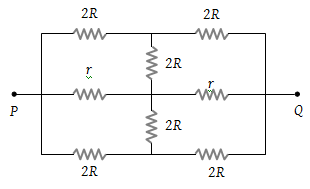NEET Physics Current Electricity Questions Solved

The effective resistance between points P and Q of the electrical circuit shown in the figure is(1) $2Rr/\left(R+r\right)$

(2) $8R\text{\hspace{0.17em}}\left(R+r\right)/\left(3R+r\right)$

(3) $2r+4R$

(4) $5R/2\text{\hspace{0.17em}\hspace{0.17em}}+\text{\hspace{0.17em}}2r$

Explanation is a part of a Paid Course. To view Explanation Please buy the course.

Difficulty Level: